Difference between revisions of "Legendre polynomials"

Legendre polynomials (aka. Legendre functions of the first kind, Legendre coefficients, or zonal harmonics) are solutions of the Legendre differential equation. The Legendre polynomial,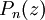$P_n (z)$ can be defined by the contour integral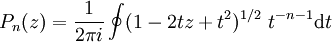$P_n (z) = \frac{1}{2 \pi i} \oint ( 1-2tz + t^2)^{1/2}~t^{-n-1} {\rm d}t$

The first seven Legendre polynomials are: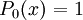$\left. P_0 (x) \right.=1$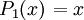$\left. P_1 (x) \right.=x$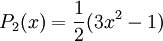$P_2 (x) =\frac{1}{2}(3x^2-1)$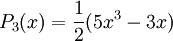$P_3 (x) =\frac{1}{2}(5x^3 -3x)$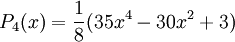$P_4 (x) =\frac{1}{8}(35x^4 - 30x^2 +3)$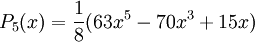$P_5 (x) =\frac{1}{8}(63x^5 - 70x^3 + 15x)$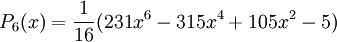$P_6 (x) =\frac{1}{16}(231x^6 -315x^4 + 105x^2 -5)$

"shifted" Legendre polynomials (which obey the orthogonality relationship):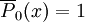$\overline{P}_0 (x) =1$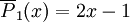$\overline{P}_1 (x) =2x -1$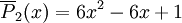$\overline{P}_2 (x) =6x^2 -6x +1$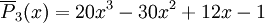$\overline{P}_3 (x) =20x^3 - 30x^2 +12x -1$

Powers in terms of Legendre polynomials: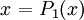$\left. x \right.= P_1 (x)$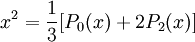$x^2= \frac{1}{3}[P_0 (x) + 2P_2(x)]$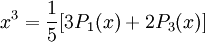$x^3= \frac{1}{5}[3P_1 (x) + 2P_3(x)]$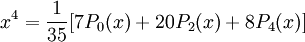$x^4= \frac{1}{35}[7P_0 (x) + 20P_2(x)+ 8P_4(x)]$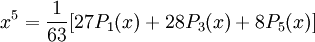$x^5= \frac{1}{63}[27P_1 (x) + 28P_3(x)+ 8P_5(x)]$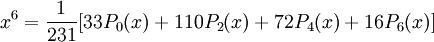$x^6= \frac{1}{231}[33P_0 (x) + 110P_2(x)+ 72P_4(x)+ 16P_6(x)]$